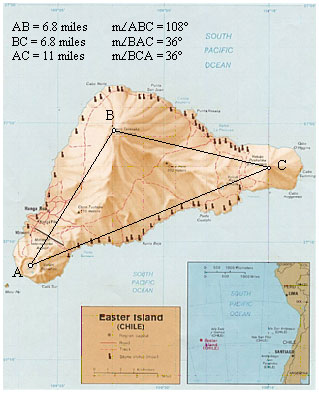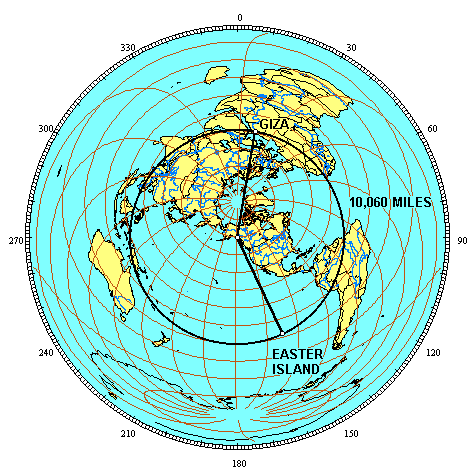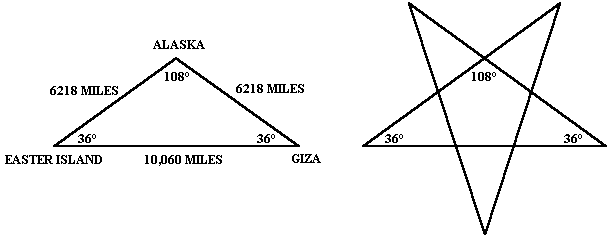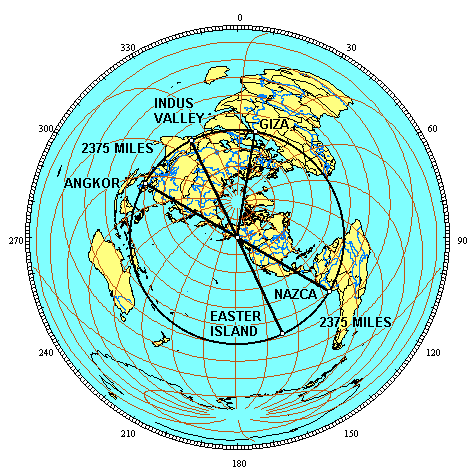View Part: 1 2 3 4 5 6 7 8 9 10 11 12

PART 6 - EASTER ISLAND

Easter Island is triangular and the three volcanic peaks on Easter form an isosceles triangle with an apex angle of 108° and base angles of 36°. The ratio between the length of the base and the lengths of the sides is φ (6.8 miles x 1.618 = 11 miles).The southern coastline is roughly parallel to the great circle as it crosses over the island. The southwestern corner of Easter is 10,060 miles from Giza. The length of the great circle segments from the axis point to Easter and to Giza is 6,218 miles.The ratio between the baselength of 10,060 miles and the side lengths of 6,218 miles is φ (6,218 x 1.618 = 10,060). The azimuth from the axis point to Giza is 9° and the azimuth from the axis point to Easter is 154°. The angle of the terrestrial triangle at the axis point is 145°.

The terrestrial angles at Easter and Giza are 90°. These terrestrial angles convert to flat surface angles of 108° at the axis point and 36° at Easter and Giza. This is the same triangle that is formed by the three volcanic peaks on Easter and the same triangle that is found in a pentagram.The φ ratio between the distance from Easter to Giza and the distances from the axis point to Easter and Giza is also shown by these measures in kilometers. One quarter of the circumference of the earth is 10,000 kilometers. The distance from Easter to Giza is 16,180 kilometers, expressing the φ ratio of 1.618 : 1.

Easter is 2375 miles from Nazca. This is 9.54% of the circumference of the earth, or 34.34°. The axis point is 6218 miles from Easter and from Nazca. The ratio between one side of the terrestrial triangle and the baselength of the triangle is 2.618 to one (2375 miles x 2.618 = 6218 miles).

The terrestrial triangle formed by Angkor Vihear, Easter’s antipodal point in the Indus Valley and the axis point has these same dimensions with the same φ² ratio between the side length and the base length. The terrestrial triangle formed by Easter’s antipodal point in the Indus Valley, Giza and the axis point has these same dimensions and the same φ² ratio.